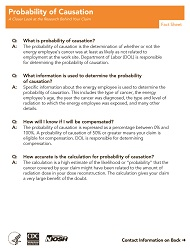# Probability of Causation

###### DHHS (NIOSH) Publication Number 2005-141This fact sheet addresses four common questions concerning the probability of causation (defined below). Q: What is probability of causation? A: The probability of causation is the determination of whether or not the energy employee’s cancer was at least as likely as not related to employment at the work site. Department of Labor (DOL) is responsible for determining the probability of causation. Q: What information is used to determine the probability of causation? A: Specific information about the energy employee is used to determine the probability of causation. This includes the type of cancer, the energy employee’s age, the year the cancer was diagnosed, the type and level of radiation to which the energy employee was exposed, and many other details. Q: How will I know if I will be compensated? A: The probability of causation is expressed as a percentage between 0% and 100%. A probability of causation of 50% or greater means your claim is eligible for compensation. DOL is responsible for determining compensation. Q: How accurate is the calculation for probability of causation? A: The calculation is a high estimate of the likelihood or “probability” that the cancer covered by your claim might have been related to the amount of radiation dose in your dose reconstruction. The calculation gives your claim a very large benefit of the doubt.

Page last reviewed: April 21, 2017